Note: The other languages of the website are Google-translated. Back to English

## Excel Formula：Calculate network time with break in a timesheet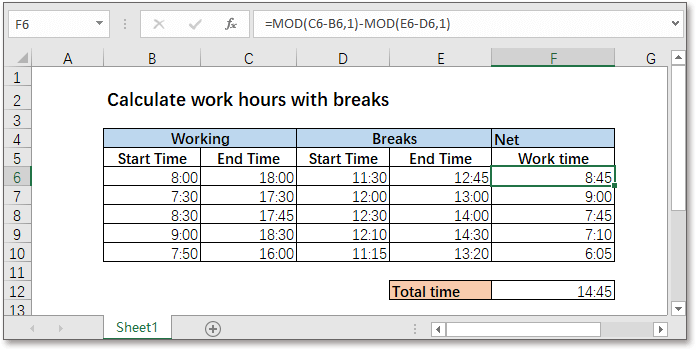Generally, while calculating the working time, the break time needed to be excluded. Here in this tutorial, it provides the formula which uses the MOD function to calculate the network time with breaks in Excel.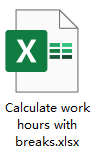Generic formula:

 MOD(work_end-work_start,1)-MOD(break_end-break_start,1)

Arguments

 Work_start: the time that starts work at. Work_end: the time that ends work at. Break_start: the time that starts break at. Break_end: the time that ends break at.

How this formula work

Here is a table containing the worktime and break time, please use below formula to calculate the network time.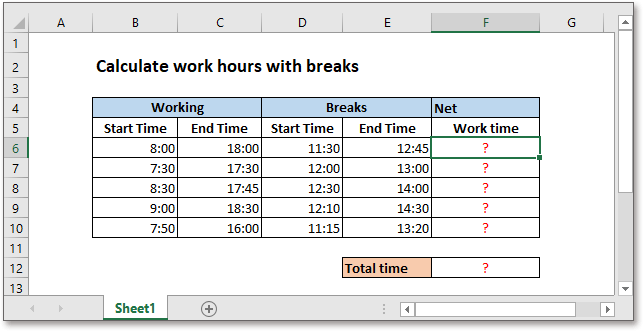=MOD(C6-B6,1)-MOD(E6-D6,1)

Press Enter key to get the network time.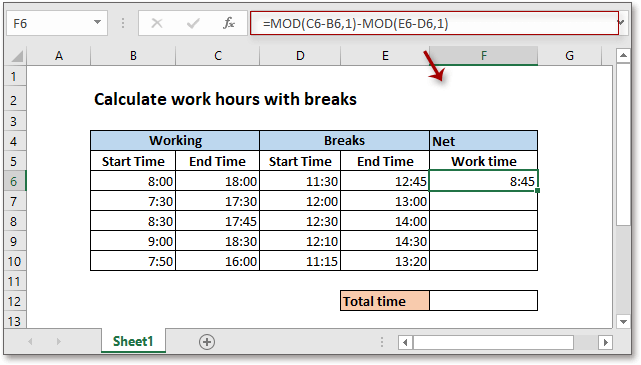Then drag autofill handle over cells to calculate the network time.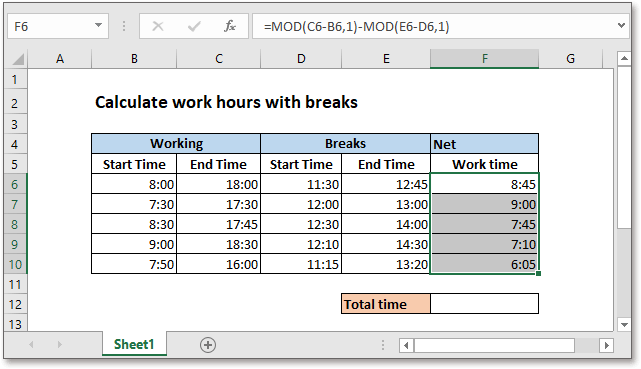Explanation

The MOD function returns the division remainder of two numbers. For example, MOD(8,3)=2.

In this case, the time value must be the decimal value, which will remain itself while dividing 1 by using MOD function.

For instance, =MOD(C6-B6,1)=10:00= 0.416666667, then =MOD(E6-D6,1)=1:15= 0.052083333. Now
=MOD(C6-B6,1)-MOD(E6-D6,1)
=10:00-1:15
=8:45

Remark

1) If you need to calculate the total network time, use the formula

=SUM(F6:F10)

Note: The sum result is in the h:mm format automatically. To understand the sum result easily, you can change it to the time format of 37:30:55.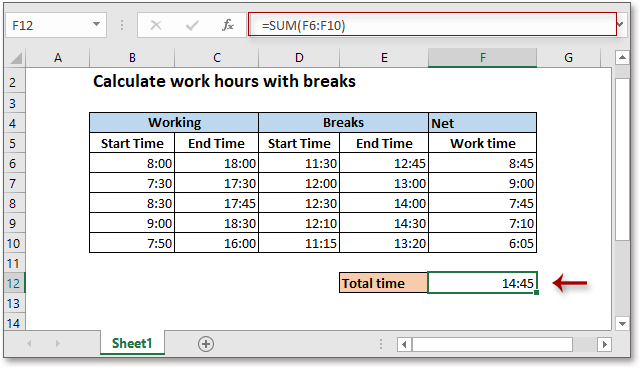2) If the timesheet records the two separate in/out times for a single shift as below show, you can use the formula as this:

MOD(work_end1-work_start1,1)-MOD(work_end2-work_start2,1)
=MOD(C17-B17,1)+MOD(E17-D17,1)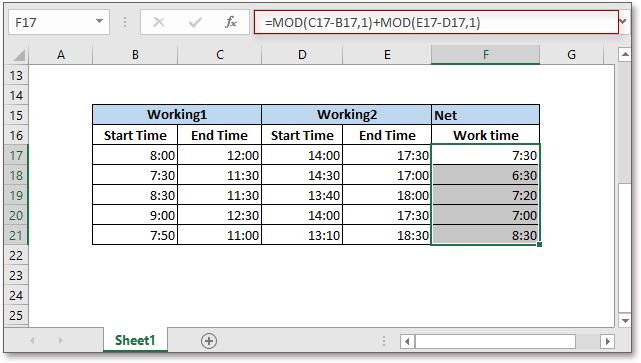### The Best Office Productivity Tools

#### Kutools for Excel - Helps You To Stand Out From Crowd

Would you like to complete your daily work quickly and perfectly? Kutools for Excel brings 300 powerful advanced features (Combine workbooks, sum by color, split cell contents, convert date, and so on...) and save 80% time for you.

• Designed for 1500 work scenarios, helps you solve 80% Excel problems.
• Reduce thousands of keyboard and mouse clicks every day, relieve your tired eyes and hands.
• Become an Excel expert in 3 minutes. No longer need to remember any painful formulas and VBA codes.
• 30-day unlimited free trial. 60-day money back guarantee. Free upgrade and support for 2 years.#### Office Tab - Enable Tabbed Reading and Editing in Microsoft Office (include Excel)

• One second to switch between dozens of open documents!
• Reduce hundreds of mouse clicks for you every day, say goodbye to mouse hand.
• Increases your productivity by 50% when viewing and editing multiple documents.
• Brings Efficient Tabs to Office (include Excel), Just Like Chrome, Firefox, And New Internet Explorer.